# Python Exception Handling

Now, we are going to spend some time learning about an interesting, and useful concept, which is Python Exception Handling.

Here is an insight into what we are going to cover –

• Python Exception Handling Introduction
• The try clause and except clause (try statement)
• Multiple except blocks for a try block
• Nesting of try, except blocks
• The finally block
• The raise keyword in exception handling
• The else block in exception handling

### Python Exception Handling Introduction

First of all, Let’s understand what an exception is. The exception is some event, which disrupts the normal flow of our program. There are certain situations when something like this may occur in our program.

For example, if we are performing a division operation, and there is 0 in the denominator, which simply means that we are dividing some number by zero, and in such cases, we get a ZeroDivisionError. Or, if we are having a list, and we are trying to access such an index, which is out of bounds, we get an IndexError. There are many such kinds of exceptions that may occur in our program. Well, the thing is that if we are getting an exception in our program, the program will abnormally terminate raising that particular exception. But this is not a good thing, since our program has crashed in this case. But the good thing is that we can handle the exceptions, and this is what we are going to have a look at now.

The thing is that the exceptions should not be confused. There are some other things as well, like the syntax error. The syntax error simply means that we have not written things correctly in our programs. Have a look at the below program, in which, we get the syntax error since we have not written the things in the way that they are supposed to be.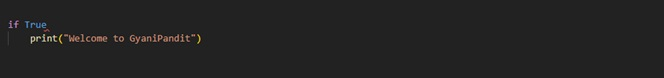As you can see, in the above program, we have written an if statement, and as the condition, we are simply giving True. After that, we intentionally forgot the colon that is supposed to be there, and then we have written some print statements. The thing is that here we are going to get an error if we try to run the program. If you try to run the program, you get a SyntaxError, which means that you have not written the things in the way that they are supposed to be. When you are learning python, you might get into such errors, from time to time.

The thing is that even if we have written things in the way that they are supposed to be, we might run into errors, while we are trying to execute them. These errors which are encountered at the time of execution are called Exceptions, and soon, we are going to learn about handling them.

There are certain things that we can do, in order to handle some of the exceptions that may occur in our program. There are many different exceptions, that can be raised in certain situations, like IndexError, FileNotFoundError, NameError, ZeroDivisionError, ValueError, OSError, and many others. We are going to have a look at what we can do to handle the exceptions, and then you can simply use them as per your requirements.

### Try clause and except clause (try statement)

When we write programs, we can simply identify the block of the code, which is raising an exception. For example, in the below example, if we are getting the ZeroDivisionError, we can identify which instruction is responsible for that exception. Have a look at the below program, which tries to demonstrate the same thing.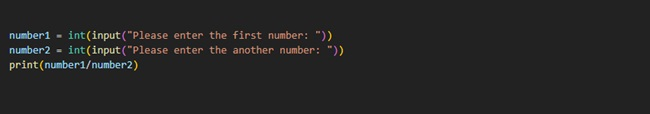As you can see in the above program, we are trying to get the numbers as user input, in order to perform division. This program, when executed, works fine most of the time when we are giving the correct inputs. But, when we put the number zero in the denominator, we get the ZeroDivisionError. So, otherwise, the program was working right (provided that the user inputs were correct), but if the denominator is 0, we get the ZeroDivisionError.

So, we just need to use the try clause, and whatever block of code that we feel can raise an exception, is to be kept in the try block. This is like we are saying – Try to execute these statements. But if some exception is raised, we have to write the corresponding except block for that. So, we use the try and except clauses here. Within the try block, we are writing the block of code, which we think may raise an exception. Also, for that particular exception, which can be raised, we are writing the handling code within the except block.

Let’s have a look at the below example, which tries to demonstrate the same thing.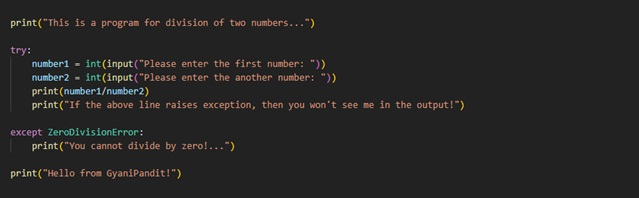As you can see, in the above program, we have written some of the instructions within the try block. This is like we are saying that you should TRY to execute these things, and if the exception is raised, there is the corresponding except block for that. So, while in the try block, if at any point, the ZeroDivisionError is raised, then we have written the corresponding except block for that. Also, remember that the except block is only going to execute if that particular exception is raised.

So, from this, we can understand that within the try block, we are writing the instructions that we think can raise some exceptions. For some particular exceptions, we can write the except block. This can be read as something like – try to execute these instructions, and if the ZeroDivisionError is raised, do this(whatever we have written in the except block for the ZeroDivisionError). Also, if you try to run the above program, you can see that the program did not terminate abnormally.

Let’s have a look at another program, where we can have another exception. Let’s consider that we are asking for a user input, which is supposed to be an integer, and the user enters something invalid, and in such case, we get a ValueError. Let’s have a look at a program, in which, we try to handle the ValueError.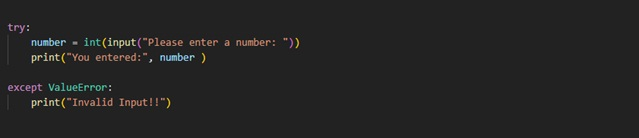As you can see, in the above program, we are asking for a number from the user. If the user enters the correct input, the number will be printed, and since the exception is not raised, the except block won’t run. But if in the program, we get the ValueError, then the except block that is written for the ValueError would execute, and we would get a message as Invalid Input! You can try executing the above program and try it for some different inputs.

### Multiple except blocks for a try block

Well, now we are familiar with the fact, that we can handle the exceptions in  Python Exception Handling, and for that, we are using the try and except blocks in our programs. You can try some more examples, including some other exceptions so that you can understand them in a clear way. Now, we are going to head to another situation. The thing is that in our program, there might be situations, when there may be the possibility of multiple exceptions in our program. Let’s have a look at the previous program again, where we tried to perform the division of two numbers.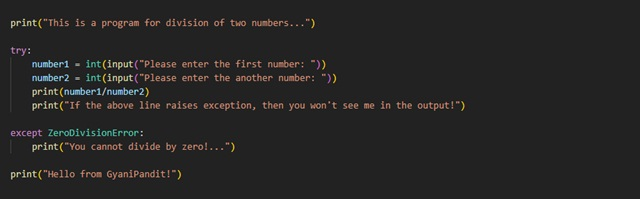In the above program, there can be more than one exception raised. The thing is that there can be a ZeroDivisionError, which we have handled, but what if the user inputs something that is not valid? In such situations, we would even get a ValueError. So, in the above program, there can be multiple exceptions. So, we can create multiple except blocks for the corresponding exceptions, with this one try block. Also, Let’s try to add one more exception, which would be the IndexError this time. Let’s have a look at the below program now.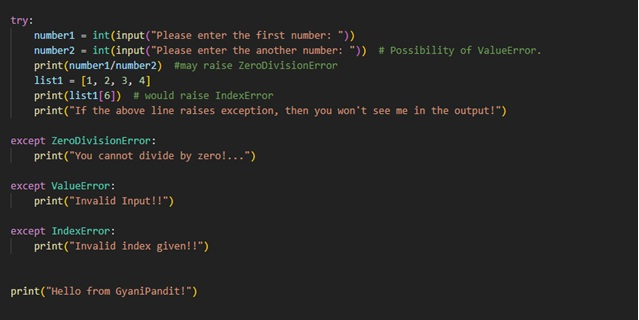As you can see, in the above program, we have a try block, within which, we have some block of code, which might raise some kind of exception. The thing is that there can be multiple exceptions coming from this block, so we have multiple except blocks here. If the block raises ValueError, immediately the except block corresponding to the ValueError would execute, and the rest of the instructions in the try block won’t execute. But at least, now the program won’t terminate abnormally.

You can try running the above program and you can see that we are getting the IndexError. But if you give some kind of invalid input, then you would get the ValueError, and the rest of the try block won’t execute. So, this way, we can understand that there can be multiple except blocks for a try block.

Python Exception Handling

### Nesting of try except blocks

We are much more familiar with the try and except blocks now. So, we are going to dive much deeper to have a look at the nesting of try-except blocks. Well, nesting simply means that we are writing try inside another try block. At times, we might require to do this, so we are going to explore how we can do this nesting. For this, we are going to have a very simple example again, in which, there would be some different instructions, which can raise some different exceptions, like the IndexError, ValueError, ZeroDivisionError, etc. Let’s have a look at the program now –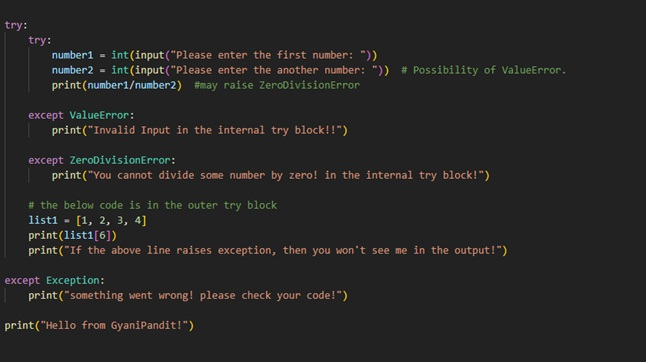The above program may seem complex at first sight, but do not worry at all, since we are going to break down this program in order to understand it.

First of all, as we can see, there is an outer try block, in which, we have an inner try block. In that inner try block, we are taking two numbers as user input (so there is a possibility of ValueError), and also, we are trying to perform a division operation on the given numbers(so there would be a possibility of ZeroDivisionError). So, for those exceptions, we have written the except blocks as well.

Also, if we come back to the outer try block, we also have a list, and then we are trying to access an invalid index of the list, due to which, we are going to have the IndexError. But for that particular exception, we have not written the except block, but there is an except block for the parent Exception, which has got the IndexError covered.

So, just understand that if there is an except block for Exception, it would have all the exceptions covered.

You can try executing the above program and have a close look at the output. Now, the thing is that if in the inner try, we have some exceptions, the related except block will execute, and we will be back in the outer try block. So, the instructions after the inner try would also execute anyways. Also, there we are trying to access some invalid index, due to which, we are going to get the IndexError, for which, the except block for Exception would execute.

So, in this way, we can simply nest the try and accept. But why would we do this? Well, just consider the below example, and let’s explain this scenario.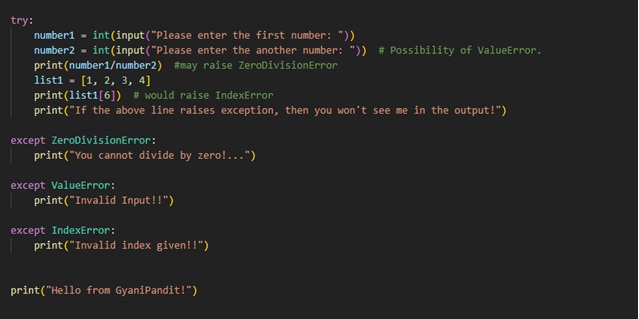You might be already familiar with this program. The thing about this program is that we are having one try block, and for that, we are having multiple except blocks. Now, Let’s say that we get an exception at the first instruction within the try block, due to which, the ValueError would be raised, and the corresponding except block would execute. But here, the rest of the try block won’t execute, due to which, nothing after that statement is executed.

But if we have all the things in a separate try block, as we did in the previous example, we will find that the other things are not getting much affected even if there is ValueError. Have a look again at the below example, which has nested try and except blocks.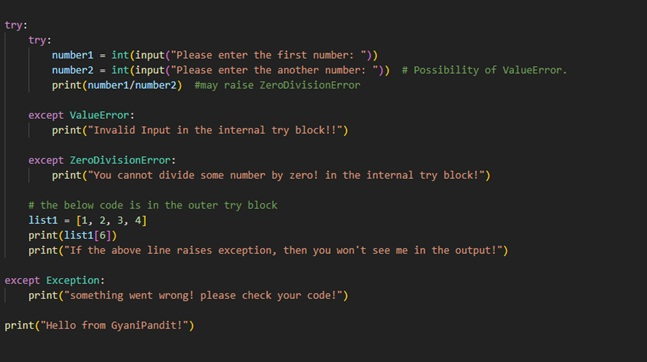In the above program, we just put the instructions for getting the user input numbers, and the instruction for performing the division of two numbers, in the inner try block. Also, we have written the except blocks for the possible exceptions raised. Now, if the exception is raised in the inner try block, then the corresponding inner except block would execute, and then we would be again in the outer try block, for doing the other things.

So, in the previous example, when there was no nesting, if ZeroDivisionError had occurred, then the code for the list would not execute, but now this is not the case. So, in such situations, it is very useful to have the nesting of try-except.

Python Exception Handling

### Finally block in Python

We have been dealing with the concept of exception handling, and so far, we have explored the try and except blocks. The code that might raise some exception is put within the try block, and the except blocks are written for corresponding exceptions with the try block, which means that if a certain exception might occur within the try block, then we would have the corresponding except block for that.

You might have observed that the except block executes only if there is some exception within the try block. Also, if there is some exception on some line in the try block, the rest of the try block won’t execute. So, do we have some place, where the code executes, whether there is an exception or no exception? Well, the finally block is the answer to this question.  Whatever we write into the finally block, would execute whether the exception was raised or not, even if the exception was raised, but not handled, the finally block would execute.

Let’s have a look at a simple program, which tries to demonstrate the finally block for us. Also, you would get a brief idea, of how and where to write the finally block.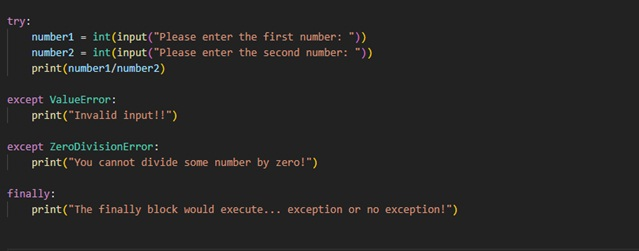As you can see, we have a simple program, for performing division of two numbers. So, within the try block, we have written the code to take the user input and perform the division of two numbers. Also, we have the corresponding except blocks for the exceptions. After that, we have written the finally block as well. The idea is that the code within the finally block is going to execute whether or not the exception is raised, or handled. You can try executing the above program and have a look at the output, for different conditions.

Now, we have mentioned that the finally block is going to execute, whether the exception is raised or not, or handled or not. This means that with a try, even if we do not write the except blocks, but we have a finally block, then even if the exception is raised, and not handled, the final block would execute, and then the program will crash. For this, you can follow the below program –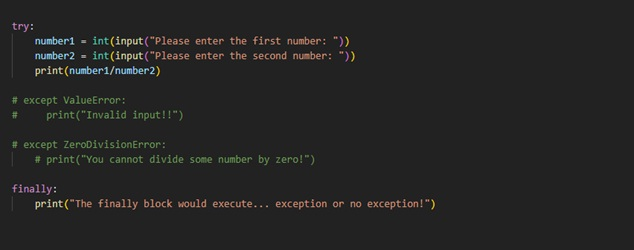As you can see, we have commented on the except blocks that were with the try block, and we have written the finally block. So now, even if the exception is raised, and it is not handled, we would find that the finally block still executes. You can execute the above program and check the output yourselves.

But now the question would be that why do we need the finally block? Well, the thing is that the finally block can be used for writing the resource cleanup codes. The thing is that if an exception is raised at some instruction, the rest try block won’t execute, and if the exception does not occur at all, then the except block won’t execute. So, we need something, where we can keep such code, which would run, even if the exception is raised or not, or handled or not, and that something is the finally block.

So, this was much about the finally block in python. You can solve some more related examples, to understand the finally block in a clearer way. You can also make use of the finally block, as and when required.

### Raise keyword in exception handling

Now, we are going to learn about the raise keyword in python. The raise keyword is basically used to raise an exception and interrupt the code execution.  We have been dealing with exception handling so far, and there might be some situations when we might want to raise some exceptions. For example, let’s say that we are creating some program, where we take a number as user input. But we want to have only the numbers greater than or equal to 0. Now, the thing is that the user can enter a negative number as well. So, in such a situation, we can raise some exceptions and interrupt the flow of the program. This is basically according to our requirements.

Let’s have a look at a simple program, in which we would do the same thing that we just discussed. We will take a number as user input, and then we would check if the number is greater than or equal to 0, then we will print some message, and if the number is less than zero, then we would raise an exception.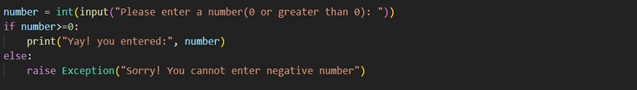As you can see in the above program, we are taking a number as user input, and then we check if the number is greater than or equal to 0. If the number is greater than or equal to zero, then we are printing some message, and else, we are raising an exception.

You can try executing the above program and try to input some negative numbers and observe the output. You would see the specified message in the exception raised.

So, as and when required, we can make use of the raise keyword in our programs. At times, when we are required to raise some exception in our program, we can make use of the raise keyword. In the above program, we are using the Exception class, but you can use some other classes related to Exception, like the ZeroDivisionError, ValueError, etc.

### Else block in exception handling in Python

The name “else” may not be new to you in python. We have used this “else” when we are dealing with certain conditions in our programs. The thing is that in many different programming languages, the “else” is usually seen with the if statement, but here in python, the “else” can also be seen in the exception handling, and now, we are going to have a look at what is the else block here in the concept of exception handling.

The thing is that when we are dealing with some conditions, the else block executes when the condition or the expression evaluates to False. But here in the case of exception handling, the else block executes, if there was no exception raised within the try block.

So, Let’s have a look at a simple program, which would demonstrate us the else block in the context of exception handling.

As you can see, this is a simple program here. We have a try block, within which, we are trying to get the two numbers as user inputs, and then we are trying to perform the division operation. Also, we are now familiar with the thing that there can be some exceptions here, like the ValueError, or the ZeroDivisionError as well. So, in the situation, if these exceptions are raised, we have got the corresponding except blocks as well. Also, below that, we have the else block, which would execute if no exception was raised while in the try. Also, in the last, we have the finally block.

So, if you try to execute the above program and have a close look at the outputs in the different cases, you would find that the else block executes if there was no exception raised. You can try to have the output for some of the different cases.

Here is a table with some of the Exceptions, and their descriptions. You can give these exceptions a try in your programs, and also try to handle them according to the requirements. Hope that you have understood the broad idea related to the concept of Exception handling. The concept is very important, not only in Python but in many other programming languages.

You can furthermore explore the exceptions, and also try some more examples, with which, you can get familiar with the concept of exception handling. Also, at times, you should be handling the exceptions from your programs, so that there won’t be some abnormal termination.